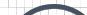Informative Information for the Uninformed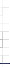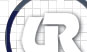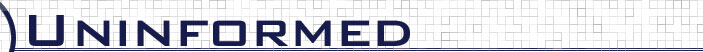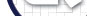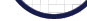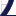Vol 5» 2006.Sep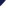# ComplexCompare Metric

Notice that the MediumCompare and SimpleCompare metrics ignore durations outside the intersection. One might think that such information would improve a fingerprinting capability, however, we found this is not the case. To illustrate, a metric called ComplexCompare was investigated. It was designed to take into account all the data points that don't fall in the intersection of two prints. ComplexCompare computes the metric that MediumCompare does and then visits every data point not in the intersection of the prints, computing duration uniqueness, or packet type duration uniqueness and then subtracting this value from the metric. The motivation for this behavior is that if L contains very unique durations and R doesn't, then the metric should be decreased proportionally by the uniqueness of these values.

Figure 4.5: ComplexCompare duration-value only analysis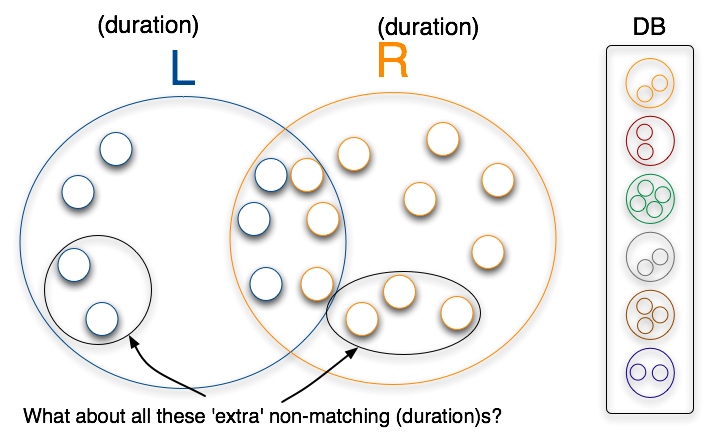ret = MediumCompare(L,R);
sum = 0;
for every duration-value d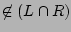sum += duration_uniqueness(d)
return ret - sum;



Figure 4.6: ComplexCompare (packet_type, duration) analysis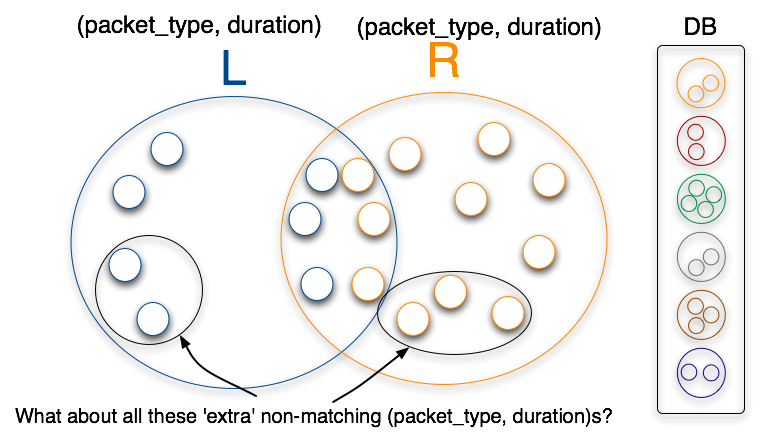ret = MediumCompare(L,R);
sum = 0;
for every packet_type p, duration-value dsum += packet_type_duration_uniqueness(p,d)
return ret - sum;